## Your friend has been given a laser for her birthday. Unfortunately, she did not receive a manual with it and so she doesn’t know the wavelen

Question

Your friend has been given a laser for her birthday. Unfortunately, she did not receive a manual with it and so she doesn’t know the wavelength that it emits. You help her by performing a double-slit experiment, with slits separated by 0.18 mmmm. You find that two adjacent bright fringes are 4.9 mmmm apart on a screen 1.4 mm from the slits.

in progress 0
6 months 2021-07-29T20:16:49+00:00 2 Answers 6 views 0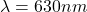Explanation:

The equation of the fringe in a double slit experiment is given by: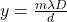(1)

here:

• y is the distance between bright fringes (two adjacent bright fringes distance = 4.9 mm).
• m is a natural number, related to the bright fringes in a diffraction pattern, in our case we use m=1
• D is the distance between the screen and the double slit (D=1.4 m)
• d is the distance between the slits (d=0.18 mm)
• λ is the wave length of the laser.

Therefor we just need to solve the equation (1) for λ: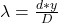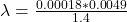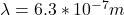I hope it helps you!

6.3*10^-7m

Explanation:

to find the wavelength of the laser you can use the following formula:y: height from the center of the screen to a fringe

m: order of the fringe

D: distance to the screen = 1.4m

d: distance between slits = 0.18mm

you know the separation of two fringes. Hence, you can use two consecutive fringes to find the wavelength in the following way: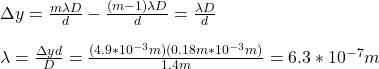hence, the walength is 6.3*10^-7m